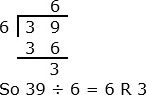# Quotient and Remainder Problem type 1 Online Quiz

Following quiz provides Multiple Choice Questions (MCQs) related to Quotient and Remainder: Problem type 1. You will have to read all the given answers and click over the correct answer. If you are not sure about the answer then you can check the answer using Show Answer button. You can use Next Quiz button to check new set of questions in the quiz.Q 1 - Divide 55 ÷ 8. Give the quotient and remainder

A - 6 R 5

B - 6 R 7

C - 7 R 3

D - 7 R 4

### Explanation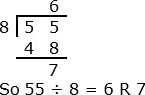Q 2 - Divide 67 ÷ 9. Give the quotient and remainder

A - 6 R 1

B - 6 R 2

C - 7 R 3

D - 7 R 4

### Explanation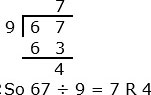Q 3 - Divide 52 ÷ 7. Give the quotient and remainder

A - 6 R 1

B - 6 R 2

C - 7 R 3

D - 7 R 4

### Explanation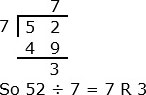Q 4 - Divide 72 ÷ 8. Give the quotient and remainder

A - 8 R 1

B - 9 R 0

C - 9 R 1

D - 9 R 4

### Explanation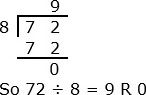Q 5 - Divide 64 ÷ 7. Give the quotient and remainder

A - 8 R 1

B - 8 R 2

C - 9 R 1

D - 9 R 4

### Explanation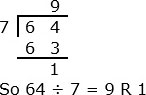Q 6 - Divide 49 ÷ 6. Give the quotient and remainder

A - 8 R 1

B - 8 R 2

C - 8 R 3

D - 8 R 4

### Explanation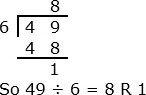Q 7 - Divide 47 ÷ 5. Give the quotient and remainder

A - 9 R 1

B - 9 R 2

C - 9 R 3

D - 9 R 4

### Explanation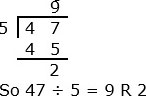Q 8 - Divide 51 ÷ 8. Give the quotient and remainder

A - 6 R 1

B - 6 R 3

C - 6 R 5

D - 6 R 7

### Explanation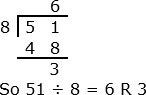Q 9 - Divide 61 ÷ 9. Give the quotient and remainder

A - 6 R 1

B - 6 R 3

C - 6 R 5

D - 6 R 7

### Explanation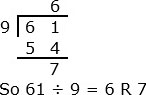Q 10 - Divide 39 ÷ 6. Give the quotient and remainder

A - 6 R 1

B - 6 R 2

C - 6 R 3

D - 6 R 4